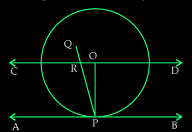Guru

# Prove that the perpendicular at the point of contact to the tangent to a circle passes through the center Q.5

• 0

How i prove the question of class 10th ncert question no.5 of exercise 10.2, give me the best and simple way to prove this question Prove that the perpendicular at the point of contact to the tangent to a circle passes through the center

Share

1. First, draw a circle with center O and draw a tangent AB which touches the radius of the circle at point P.

To Proof: PQ passes through point O.

Now, let us consider that PQ doesn’t pass through point O. Also, draw a CD parallel to AB through O. Here, CD is a straight line and AB is the tangent. Refer the diagram now.From the above diagram, PQ intersects CD and AB at R and P respectively.

As, CD ∥ AB,

Here, the line segment PQ is the line of intersection.

Now angles ORP and RPA are equal as they are alternate interior angles

So, ∠ORP = ∠RPA

And,

∠RPA = 90° (Since, PQ is perpendicular to AB)

∠ORP = 90°

Now, ∠ROP+∠OPA = 180° (Since they are co-interior angles)

∠ROP+90° = 180°

∠ROP = 90°

Now, it is seen that the △ORP has two right angles which are ∠ORP and ∠ROP. Since this condition is impossible, it can be said the supposition we took is wrong.

• -1